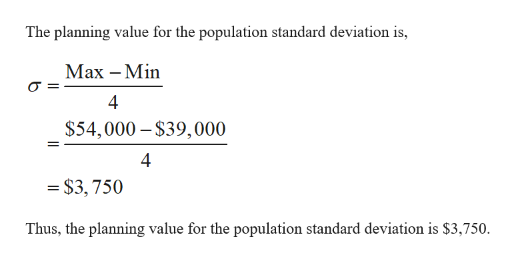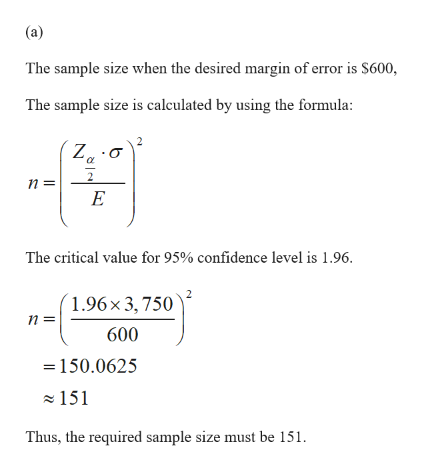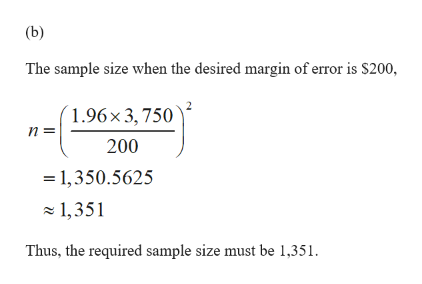# Annual starting salaries for college graduates with degrees in business administration are generally expected to be between \$39,000 and \$54,000. Assume that a 95% confidence interval estimate of the population mean annual starting salary is desired. (Round your answers up to the nearest whole number.)What is the planning value for the population standard deviation? (a)How large a sample should be taken if the desired margin of error is \$600? (b)How large a sample should be taken if the desired margin of error is \$200? (c)How large a sample should be taken if the desired margin of error is \$100? (d)Would you recommend trying to obtain the \$100 margin of error? Explain.

Question
2 views
Annual starting salaries for college graduates with degrees in business administration are generally expected to be between \$39,000 and \$54,000. Assume that a 95% confidence interval estimate of the population mean annual starting salary is desired. (Round your answers up to the nearest whole number.)
What is the planning value for the population standard deviation?

(a)
How large a sample should be taken if the desired margin of error is \$600?

(b)
How large a sample should be taken if the desired margin of error is \$200?

(c)
How large a sample should be taken if the desired margin of error is \$100?

(d)
Would you recommend trying to obtain the \$100 margin of error? Explain.

check_circle

Step 1help_outlineImage TranscriptioncloseThe planning value for the population standard deviation is, Мах — Min 4 \$54,000 – \$39,000 4 = \$3, 750 Thus, the planning value for the population standard deviation is \$3,750. fullscreen
Step 2help_outlineImage Transcriptionclose(a) The sample size when the desired margin of error is \$600, The sample size is calculated by using the formula: Z,:0 The critical value for 95% confidence level is 1.96. (1.96× 3,750 600 =150.0625 z 151 Thus, the required sample size must be 151. fullscreen
Step 3help_outlineImage Transcriptionclose(b) The sample size when the desired margin of error is S200, (1.96×3,750 п 200 = 1,350.5625 z 1,351 Thus, the required sample size must be 1,351. fullscreen

### Want to see the full answer?

See Solution

#### Want to see this answer and more?

Solutions are written by subject experts who are available 24/7. Questions are typically answered within 1 hour.*

See Solution
*Response times may vary by subject and question.
Tagged in